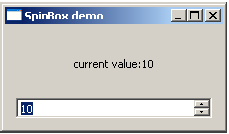PyQt - QSpinBox Widget

A QSpinBox object presents the user with a textbox which displays an integer with up/down button on its right. The value in the textbox increases/decreases if the up/down button is pressed.

By default, the integer number in the box starts with 0, goes upto 99 and changes by step 1. Use QDoubleSpinBox for float values.

Important methods of QSpinBox class are listed in the following table −

Sr.No. Methods & Description
1

setMinimum()

Sets the lower bound of counter

2

setMaximum()

Sets the upper bound of counter

3

setRange()

Sets the minimum, maximum and step value

4

setValue()

Sets the value of spin box programmatically

5

Value()

Returns the current value

6

singleStep()

Sets the step value of counter

QSpinBox object emits valueChanged() signal every time when up/own button is pressed. The associated slot function can retrieve current value of the widget by value() method.

Following example has a label (l1) and spinbox (sp) put in vertical layout of a top window. The valueChanged() signal is connected to valuechange() method.

self.sp.valueChanged.connect(self.valuechange)

The valueChange() function displays the current value as caption of the label.

self.l1.setText("current value:"+str(self.sp.value()))

The complete code is as follows −

import sys
from PyQt4.QtCore import *
from PyQt4.QtGui import *

class spindemo(QWidget):
def __init__(self, parent = None):
super(spindemo, self).__init__(parent)

layout = QVBoxLayout()
self.l1 = QLabel("current value:")
self.l1.setAlignment(Qt.AlignCenter)
self.sp = QSpinBox()

self.sp.valueChanged.connect(self.valuechange)
self.setLayout(layout)
self.setWindowTitle("SpinBox demo")

def valuechange(self):
self.l1.setText("current value:"+str(self.sp.value()))

def main():
app = QApplication(sys.argv)
ex = spindemo()
ex.show()
sys.exit(app.exec_())

if __name__ == '__main__':
main()

The above code produces the following output −